# 相对论物质波及其对超导电性的解释：基于平等性原理Relativistic Matter Wave and Its Explanation to Superconductivity: Based on the Equality Principle

DOI: 10.12677/MP.2020.103005, PDF, HTML, XML, 下载: 268  浏览: 1,231

Abstract: Relativistic matter waves provide a basic concept for the study of quantum strongly correlated systems in superconductors. In this paper, by studying the phase change of relativistic matter wave in the process of electron collision, the interaction formula of strongly correlated electron system is derived, and its validity in superconductor is checked. Our calculation of energy gap agrees well with experiments for 21 typical superconductors. Moreover, this formula clearly indicates that some electrons colliding with crystal lattice will become no gain and no loss in energy at extremely low temperatures. It provides a new way to study the mechanism of superconductivity. In addition, we put forward that relativistic matter wave is a basic concept based on the equality principle, which makes our superconductivity theory have a more solid theoretical foundation.

1. 引言

2. 粒子碰撞中的相对论物质波

$\varphi =\mathrm{exp}\left(\frac{i}{\hslash }{\int }_{{x}_{0}\left(L\right)}^{x}\left(m{u}_{\mu }+q{A}_{\mu }\right)\text{d}{x}_{\mu }\right)\text{ }⇔\text{ }{\stackrel{^}{p}}_{\mu }\equiv -i\hslash \frac{\partial }{\partial {x}_{\mu }}-q{A}_{\mu }$ . (1)

$\begin{array}{l}{\varphi }^{\left(1\right)}=\mathrm{exp}\left(\frac{i}{\hslash }{\int }_{{x}_{0}\left(L\right)}^{x}\left({p}_{\mu }^{\left(1\right)}+{q}^{\left(1\right)}{A}_{\mu }^{\left(1\right)}\right)\text{d}{x}_{\mu }\right)=\mathrm{exp}\left(\frac{i}{\hslash }{\int }_{{x}_{0}\left(L\right)}^{x}{R}_{\mu }^{\left(1\right)}\text{d}{x}_{\mu }\right)\\ {\varphi }^{\left(2\right)}=\mathrm{exp}\left(\frac{i}{\hslash }{\int }_{{x}_{0}\left(L\right)}^{x}\left({p}_{\mu }^{\left(2\right)}+{q}^{\left(2\right)}{A}_{\mu }^{\left(2\right)}\right)\text{d}{x}_{\mu }\right)=\mathrm{exp}\left(\frac{i}{\hslash }{\int }_{{x}_{0}\left(L\right)}^{x}{R}_{\mu }^{\left(2\right)}\text{d}{x}_{\mu }\right)\end{array}$ , (2)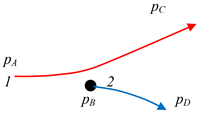Figure 1. Particle 1 collides off particle 2

$\begin{array}{l}{A}_{\mu }^{\left(1\right)}=\frac{1}{4\pi {\epsilon }_{0}}\frac{{q}^{\left(2\right)}{u}_{\mu }^{\left(2\right)}}{{c}^{2}r}\propto {p}_{\mu }^{\left(2\right)}\\ {A}_{\mu }^{\left(2\right)}=\frac{1}{4\pi {\epsilon }_{0}}\frac{{q}^{\left(1\right)}{u}_{\mu }^{\left(1\right)}}{{c}^{2}r}\propto {p}_{\mu }^{\left(1\right)}\end{array}$ . (3)

$\begin{array}{l}\left[\begin{array}{c}{R}_{\mu }^{\left(1\right)}\\ {R}_{\mu }^{\left(2\right)}\end{array}\right]=\left(1+\left[\begin{array}{cc}{S}^{\left(11\right)}& {S}^{\left(12\right)}\\ {S}^{\left(21\right)}& {S}^{\left(22\right)}\end{array}\right]\right)\left[\begin{array}{c}{p}_{\mu }^{\left(1\right)}\\ {p}_{\mu }^{\left(2\right)}\end{array}\right]\\ \text{or}\text{ }R=\left(1+S\right)p\end{array}$ . (4)

$\left[\begin{array}{c}{R}^{\left(1\right)}\\ {R}^{\left(2\right)}\end{array}\right]=\left(1+S\right)\left[\begin{array}{c}{p}^{\left(1\right)}\\ {p}^{\left(2\right)}\end{array}\right]=\left(1+{c}_{1}{\sigma }_{1}+{c}_{2}{\sigma }_{2}+{c}_{3}{\sigma }_{3}\right)\left[\begin{array}{c}{p}^{\left(1\right)}\\ {p}^{\left(2\right)}\end{array}\right]$ ,(5)

${\sigma }_{1}=\left[\begin{array}{cc}0& 1\\ 1& 0\end{array}\right],\text{ }{\sigma }_{2}=\left[\begin{array}{cc}0& -i\\ i& 0\end{array}\right],\text{ }{\sigma }_{3}=\left[\begin{array}{cc}1& 0\\ 0& -1\end{array}\right]$ , (6)

1) 假设在碰撞过程中c1工作， ${c}_{\text{2}}={c}_{\text{3}}=0$ ，这种情况是

$\left[\begin{array}{c}{R}_{\mu }^{\left(1\right)}\\ {R}_{\mu }^{\left(2\right)}\end{array}\right]=\left(1+{c}_{1}\left[\begin{array}{cc}0& 1\\ 1& 0\end{array}\right]\right)\left[\begin{array}{c}{p}_{\mu }^{\left(1\right)}\\ {p}_{\mu }^{\left(2\right)}\end{array}\right]$ .(7)

$\left[\begin{array}{c}{R}_{\mu }^{\left(1\right)}\\ {R}_{\mu }^{\left(2\right)}\end{array}\right]=\left[\begin{array}{c}{p}_{\mu }^{\left(1\right)}\\ {p}_{\mu }^{\left(2\right)}\end{array}\right]+\left[\begin{array}{c}{q}^{\left(1\right)}{A}_{\mu }^{\left(1\right)}\\ {q}^{\left(2\right)}{A}_{\mu }^{\left(2\right)}\end{array}\right]$ . (8)

2) 假设在碰撞过程中c1和c3工作， ${c}_{\text{2}}=0$ ，这种情况是

$\begin{array}{c}\left[\begin{array}{c}{R}_{\mu }^{\left(1\right)}\\ {R}_{\mu }^{\left(2\right)}\end{array}\right]=\left(1+{c}_{1}\left[\begin{array}{cc}0& 1\\ 1& 0\end{array}\right]+{c}_{3}\left[\begin{array}{cc}1& 0\\ 0& -1\end{array}\right]\right)\left[\begin{array}{c}{p}_{\mu }^{\left(1\right)}\\ {p}_{\mu }^{\left(2\right)}\end{array}\right]\\ =\left(1+{c}_{3}\left[\begin{array}{cc}1& 0\\ 0& -1\end{array}\right]\right)\left[\begin{array}{c}{p}_{\mu }^{\left(1\right)}\\ {p}_{\mu }^{\left(2\right)}\end{array}\right]+\left[\begin{array}{c}{q}^{\left(1\right)}{A}_{\mu }^{\left(1\right)}\\ {q}^{\left(2\right)}{A}_{\mu }^{\left(2\right)}\end{array}\right]\end{array}$ .(9)

$\begin{array}{l}{R}_{4}={R}_{4}^{\left(1\right)}+{R}_{4}^{\left(2\right)}\\ R={R}^{\left(1\right)}+{R}^{\left(2\right)}\end{array}$ .(10)

$\begin{array}{l}{R}_{4}={p}_{4}^{\left(1\right)}+{c}_{3}{p}_{4}^{\left(1\right)}+{q}^{\left(1\right)}{A}_{4}^{\left(1\right)}+{p}_{4}^{\left(2\right)}-{c}_{3}{p}_{4}^{\left(2\right)}+{q}^{\left(2\right)}{A}_{4}^{\left(2\right)}\\ R={p}^{\left(1\right)}+{c}_{3}{p}^{\left(1\right)}+{q}^{\left(1\right)}{A}^{\left(1\right)}+{p}^{\left(2\right)}-{c}_{3}{p}^{\left(2\right)}+{q}^{\left(2\right)}{A}^{\left(2\right)}\end{array}$ . (11)

${c}_{3}=\frac{{p}_{4}^{\left(1\right)}+{q}^{\left(1\right)}{A}_{4}^{\left(1\right)}+{p}_{4}^{\left(2\right)}+{q}^{\left(2\right)}{A}_{4}^{\left(2\right)}-{R}_{4}}{{p}_{4}^{\left(2\right)}-{p}_{4}^{\left(1\right)}}$ , (12)

${c}_{3}=\frac{|{p}^{\left(1\right)}+{q}^{\left(1\right)}{A}^{\left(1\right)}+{p}^{\left(2\right)}+{q}^{\left(2\right)}{A}^{\left(2\right)}-R|}{|{p}^{\left(2\right)}-{p}^{\left(1\right)}|}$ . (13)

${p}_{4}=\frac{mic}{\sqrt{1-{v}^{2}/{c}^{2}}}=\frac{i{E}_{r}}{c};\text{ }{p}_{4}^{\left(2\right)}\ne {p}_{4}^{\left(1\right)};\text{ }{p}^{\left(2\right)}\ne {p}^{\left(1\right)}$ . (14)

${\varphi }^{\left(1\right)}{\varphi }^{\left(2\right)}=\varphi \left({x}^{\left(1\right)},{x}^{\left(2\right)}\right)\mathrm{exp}\left(-\frac{iE}{\hslash }t\right)$ ,(15)

$\begin{array}{c}{\varphi }^{\left(1\right)}{\varphi }^{\left(2\right)}=\mathrm{exp}\left(\frac{i}{\hslash }{\int }_{{x}_{0}\left(L\right)}^{x}{R}_{\mu }^{\left(1\right)}\text{d}{x}_{\mu }+\frac{i}{\hslash }{\int }_{{x}_{0}\left(L\right)}^{x}{R}_{\mu }^{\left(2\right)}\text{d}{x}_{\mu }\right)\\ =\mathrm{exp}\left(\frac{i}{\hslash }{\int }_{{x}_{0}\left(L\right)}^{x}{R}^{\left(1\right)}\cdot \text{d}{x}^{\left(1\right)}+\frac{i}{\hslash }{\int }_{{x}_{0}\left(L\right)}^{x}{R}^{\left(2\right)}\cdot \text{d}{x}^{\left(2\right)}+\frac{i}{\hslash }{\int }_{{x}_{0}\left(L\right)}^{x}\left({R}_{4}^{\left(1\right)}+{R}_{4}^{\left(2\right)}\right)\text{d}{x}_{4}\right)\end{array}$ , (16)

${R}_{4}={R}_{4}^{\left(1\right)}+{R}_{4}^{\left(2\right)}=\frac{iE}{c}=const.$ .(17)

${q}^{\left(1\right)}{A}_{4}^{\left(1\right)}+{q}^{\left(2\right)}{A}_{4}^{\left(2\right)}=\frac{1}{4\pi {\epsilon }_{0}}\frac{{q}^{\left(1\right)}{q}^{\left(2\right)}{u}_{4}^{\left(2\right)}}{{c}^{2}r}+\frac{1}{4\pi {\epsilon }_{0}}\frac{{q}^{\left(1\right)}{q}^{\left(2\right)}{u}_{4}^{\left(1\right)}}{{c}^{2}r}\propto \frac{1}{r}$ ,(18)

${c}_{3}=\frac{H\left(T,1/r\right)}{{p}_{4}^{\left(2\right)}-{p}_{4}^{\left(1\right)}}+\frac{M\left(T,1/r\right)}{|{p}^{\left(2\right)}-{p}^{\left(1\right)}|}$ , (19)

${R}_{\mu }^{\left(1\right)}={p}_{\mu }^{\left(1\right)}+\left(\frac{H\left(T,1/r\right)}{{p}_{4}^{\left(2\right)}-{p}_{4}^{\left(1\right)}}+\frac{M\left(T,1/r\right)}{|{p}^{\left(2\right)}-{p}^{\left(1\right)}|}\right){p}_{\mu }^{\left(\text{1}\right)}+{q}^{\left(1\right)}{A}_{\mu }^{\left(1\right)}$ . (20). (21)

3) 假设在碰撞过程中c1和c2工作，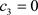，这种情况是. (22)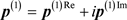. (23)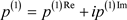. (24)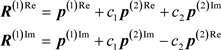. (25)

3. 相对论物质波对超导电性的解释, (26)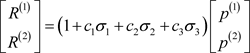. (27). (28)Figure 2. Electronenergy level distribution near the Fermi energy in the electron gas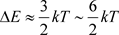. (29)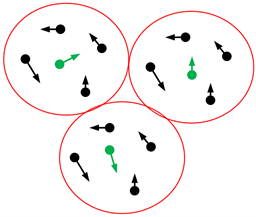Figure 3. Only a few E2 electrons become local leaders bullying over other neighboring electrons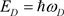. (30)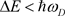. (31). (32)Table 1. Comparison of energy gaps for 21 typical superconductors

4. 相对论物质波的概念基础：平等性原理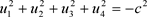. (33)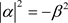. (34)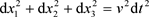. (35)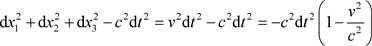. (36)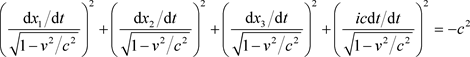. (37)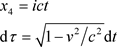. (38). (39). (40)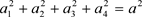. (41)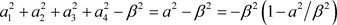. (42). (43). (44)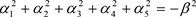. (45)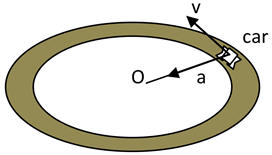Figure 4. A car moves around a circular path at constant speed v with constant centripetal acceleration a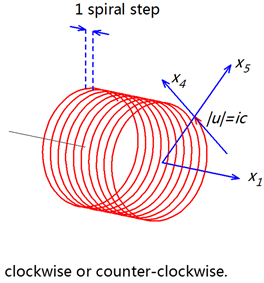Figure 5. The electron moves along the x1 axis with the constant speed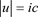in the u direction and constant centripetal force in the x5 axis at the radius iR (imaginary number), the coordinate system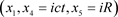consists of a cylinder coordinate system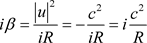. (46). (47)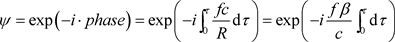. (48). (49)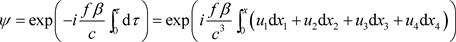. (50). (51)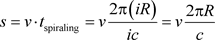. (52). (53)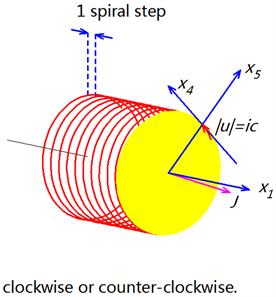Figure 6. The direction of the angular momentum J would be different from the advance x1 direction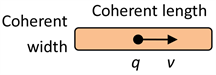Figure 7. The length of spiral-package is recognized as the coherent length of its matter wave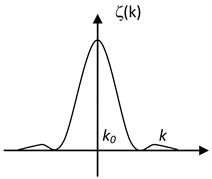Figure 8. The component spectrum is a sharp about k0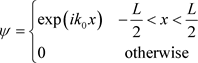. (54)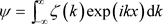. (55). (56). (57)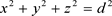. (58)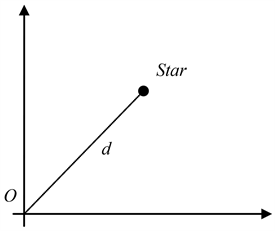Figure 9. Afar star in the sun reference frame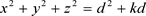. (59)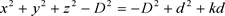. (60). (61). (62)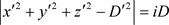. (63). (64)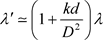. (65)

5. 结论, (A1), (A2). (A3)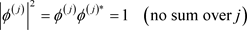. (A4), (A5). (A6)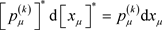. (A7)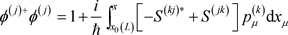. (A8)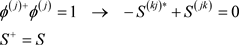. (A9)

  Poole Jr., C.P. and Farach, H.A. (2007) Superconductivity. 2nd Edition, Elsevier, Amsterdam. https://doi.org/10.1016/B978-012088761-3/50034-X  Annett, J.F. (2004) Superconductivity, Sperfluids and Condensates. Oxford University Press, Oxford.  Parsons, M.F., Mazurenko, A., Chiu, C.S., et al. (2016) Site-Resolved Measurement of the Spin-Correlation Function in the Fermi-Hubbard Mode. Science, 353, 1253-1256. https://doi.org/10.1126/science.aag1430  Boll, M., Hilker, T.A., Salomon, G., et al. (2016) Spin- and Density-Resolved Microscopy of Antiferromagnetic Correlations in Fermi-Hubbard Chains. Science, 353, 1257-1260. https://doi.org/10.1126/science.aag1635  Cheuk, L.W., Nichols, M.A., Lawrence, K.R., et al. (2016) Ob-servation of Spatial Charge and Spin Correlations in the 2D Fermi-Hubbard Model. Science, 353, 1260-1264. https://doi.org/10.1126/science.aag1635  Shen, Y., Li, Y.D., Wo, H.L., et al. (2016) Evidence for a Spinon Fermi Surface in a Triangular-Lattice Quantum-Spin-Liquid Candidate. Nature, 540, 559-562. https://doi.org/10.1038/nature20614  崔怀洋. 相对论的德布罗意物质波及其应用于Aharonov-Bohm效应[J]. 大学物理, 2017, 36 (8): 1-4+35.  Harris, E.G. (1975) Introduction to Modern Theoretical Physics. John Wiley & Sons, 266. https://doi.org/10.1007/BF00207145  Cui, H.Y. (2018) Relativistic Matterwave Contains SU(n) Symmetry. https://arxiv.org/pdf/1806.02159  崔怀洋. 相对论的德布罗意物质波及其应用于量子跃迁Rabi振荡过程[J]. 现代物理, 2018, 8(3): 139-147. https://doi.org/10.12677/MP.2018.83017  崔怀洋. 四维速度与四维力的正交性及其在教学中的应用[J]. 现代物理, 2018, 8(3): 132-138.